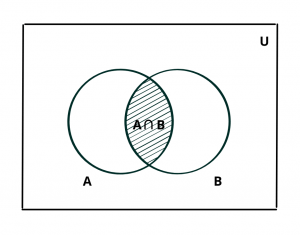# Intersection of Sets – Definition and Venn Diagram with Examples

Here you will learn what is the intersection of sets with definition and venn diagram and examples.

Let’s begin –

## What is the Intersection of Sets ?

Definition : Let A and B be two sets. The intersection of A and B is the set of all those elements that belong to both A and B.

The intersection of A and B is denoted by $$A \cap B$$ (read as “A intersection B”)Thus, $$A \cap B$$ = {x : x $$\in$$ A and x $$\in$$ B}.

Clearly, x $$\in$$ $$A \cap B$$  $$\iff$$  x $$\in$$ A and x $$\in$$ B.

In the given figure, the shaded region represents $$A \cap B$$. This is the venn diagram for intersection of sets.

Evidently, $$A \cap B$$ $$\subseteq$$ A, $$A \cap B$$ $$\subseteq$$ B.

Note : If $$A_1$$, $$A_2$$, …. , $$A_n$$ is a finite family of sets, then their intersection is denoted by $$A_1 \cap A_2 \cap ….. \cap A_n$$.

Example : If A = {1, 2, 3, 4, 5} and B = {1, 3, 9, 12}, then $$A \cap B$$ = {1, 3}.

Example : If A = {1, 2, 3, 4, 5, 6, 7}, B = {2, 4, 6, 8} and C = {4, 6, 7, 8, 9, 10, 11}, then find $$A \cap B$$ and $$A \cap B \cap C$$.

Solution : $$A \cap B$$ = {2, 4, 6}

$$\therefore$$  $$A \cap B \cap C$$ = {4, 6}

## Formula to Find Number of Elements in A Intersection B

If A, B and C are finite sets, and U be the finite universal set, then

n($$A \cap B$$) = n(A) + n(B) – n($$A \cup B$$)

where, n(A) = number of elements in set A

n(B) = number of elements in set B

n($$A \cup B$$) = number of elements in union of sets A and B

Also Read : Other Formulas and Operation of Sets

Example : If X and Y are two sets such that n(X) = 17, n(Y) = 23 and n($$X \cup Y$$) = 38, then find n($$X \cap Y$$).

Solution : By using the above formula,

n($$X \cap Y$$) = n(X) + n(Y) – n($$X \cup Y$$)

$$\implies$$  n($$X \cap Y$$) = 17 + 23 – 38

$$\implies$$  n($$X \cap Y$$) = 2